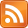SMS scnews item created by Daniel Daners at Fri 5 Jul 2019 1349
Type: Seminar
Distribution: World
Expiry: 10 Jul 2019
Calendar1: 10 Jul 2019 1400-1500
CalLoc1: AGR Carslaw 829
CalTitle1: Sickel: The interplay of smoothness and regularity in case of radial functions
Auth: daners@dora.maths.usyd.edu.au

# The interplay of smoothness and regularity in case of radial functions

### Sickel

Winfried Sickel
Friedrich-Schiller-Universität Jena, Germany
Wed 10th Jul 2019, 2-3pm, Carslaw Room 829 (AGR)

## Abstract

At the end of the seventies Strauss was the first who observed that there is an interplay between the regularity and decay properties of radial functions. We recall his

Radial Lemma: Let $d\ge 2$. Every radial function $f\in {H}^{1}\left({R}^{d}\right)$ is almost everywhere equal to a function $\stackrel{̃}{f}$, continuous for $x\ne 0$, such that

 $|\stackrel{̃}{f}\left(x\right)|\le c|x{|}^{\left(1-d\right)∕2}\parallel f\mid {H}^{1}\left({R}^{d}\right)\parallel ,\phantom{\rule{2em}{0ex}}x\ne 0,$ (1)

where $c$ depends only on $d$.

Strauss stated (1) with the extra condition $|x|\ge 1$, but this restriction is not needed.

The Radial Lemma contains three different assertions:

(a) the existence of a representative of $f$ , which is continuous outside the origin;

(b) the decay of $f$ near infinity;

(c) the limited unboundedness near the origin.

These three properties do not extend to all functions in ${H}^{1}\left({ℝ}^{d}\right)$, of course. In particular, ${H}^{1}\left({ℝ}^{d}\right)⊈{L}^{\infty }\left({R}^{d}\right)$, $d\ge 2$, and consequently, functions in ${H}^{1}\left({ℝ}^{d}\right)$ can be unbounded in the neigborhood of any fixed point $x\in {ℝ}^{d}$. The decay properties of radial functions can be used to prove compactness of embeddings of radial subspaces into Lebesgue spaces. Let $R{H}^{1}\left({ℝ}^{d}\right)$ denote the subspace of ${H}^{1}\left({ℝ}^{d}\right)$ consisting of all radial functions in ${H}^{1}\left({ℝ}^{d}\right)$. Then

$R{H}^{1}\left({ℝ}^{d}\right)↪↪{L}^{p}\left({ℝ}^{d}\right)$

holds, if $2 , where ${q}^{*}:=\infty$ if $d=2$ and ${q}^{*}=2d∕\left(d-2\right)$ if $d\ge 3$.

We will give a survey how these classical results extend to functions spaces with fractional order of smoothness like Besov and Lizorkin-Triebel spaces.

For Seminar announcements you can now subscribe to theSeminar RSS feed. Check also the PDE Seminar page.

Enquiries to Daniel Hauer or Daniel Daners.

Actions:Calendar (ICS file) download, for import into your favourite calendar applicationUNCLUTTER for printingAUTHENTICATE to mark the scnews item as read
School members may try to .